# Excel Spreadsheet Templates for Manning Equation Calculations with Partially Full Pipe Flow

Page content

## Approaches to Partially Full Pipe Flow Calculations

Partially full pipe flow under gravity is an example of open channel flow, so the Manning equation [Q = (1.49/n)A(R2/3)(S1/2)] applies if it is uniform flow. For background on the Manning Equation, see the article, “Introduction to the Manning Equation for Uniform Open Channel Flow Calculations.” A difficulty

with the use of the Manning equation, however, is the complicated nature of the equations for area of flow and wetted perimeter for partially full pipe flow. As a result there is no relatively simple equation for hydraulic radius in terms of flow depth and pipe diameter. This has led to the use of graphs of Q/Qfull and V/Vfull vs y/D, like the one shown at the left. Q and V are the flow rate and velocity with depth of flow y in a pipe of diameter D. Qfull and Vfull can be readily calculated from the Manning equation, because the hydraulic radius for a circular pipe flowing full is simply D/4.

Excel formulas, however, make use of the rather inconvenient equations for partially full pipe flow easy to use. Four downloadable Excel spreadsheet templates for making different types of partially full pipe flow calculations are presented and discussed in the rest of this article.

## The Manning Roughness Coefficient and Partially Full Pipe Flow

The cross-sectional area, wetted perimeter, and hydraulic radius for partially full pipe flow can be calculated for given pipe diameter and depth of flow with the geometric/trigonometric equations that are discussed in the next couple of sections. If the pipe slope and Manning roughness coefficient are known, then the Manning equation can be conveniently used to calculate flow rate and velocity for the given depth of flow. Unfortunately there is a flaw in this procedure. By the mid twentieth century it had been observed that measured flow rates in partially full pipe flow don’t agree with those calculated as just described. T.R. Camp developed a method for improving the agreement of partially full pipe flow calculations with measured values and presented it in a 1946 journal article (ref #2). Camps method consisted of using a variation in Manning roughness coefficient with depth of flow as shown in the graph in the preceding section.

Intuitively, one would not expect the Manning roughness coefficient to change due to a change in depth of flow in a pipe. It is well to keep in mind, however, that the Manning equation is an empirical equation. It was developed for, and is widely used with great success for, flow in rectangular and trapezoidal channels using a constant value for the Manning roughness coefficient, n. For partially full pipe flow, however, use of the variation in Manning roughness coefficient, developed by Camp and shown in the diagram above, gives better agreement with experimental measurements.

If you are making partially full pipe flow calculations, you should be aware that there are online calculators and websites with equations for making partially full pipe flow calculations using the Manning equation with constant Manning roughness coefficient, n. The equations and spreadsheets presented and discussed in this article use the variation in n that was developed by T.R. Camp.

Go to page 2 for discussion of Excel spreadsheet templates and the equations used in them for calculating Q and V in partially full pipe flow.

## Excel Template for Calculation of Q and V with Pipe Less Than Half Full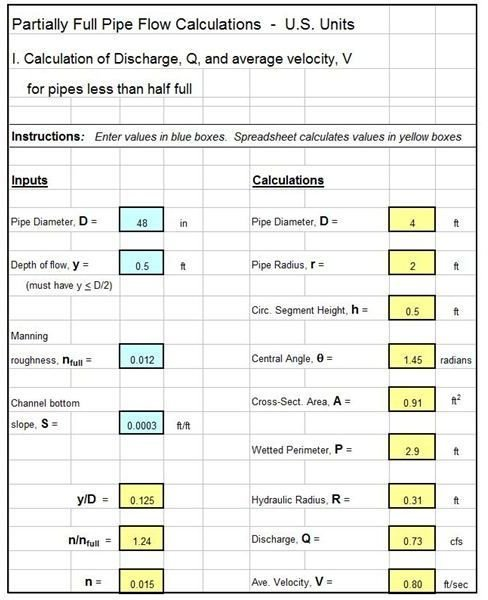The Excel template shown at the left can be used to calculate the volumetric flow rate, Q, and flow velocity, V,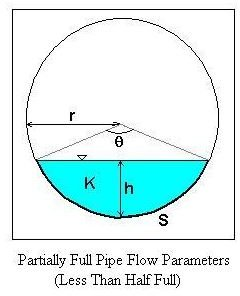for specified pipe diameter, D; flow depth, y; Manning roughness for full pipe flow, nfull; and bottom slope, S, for a partially full pipe that is flowing less than half full.

The diagram at the right shows the parameters used in partially full pipe flow calculations when the flow depth is less than the pipe radius. K is the circular segment area; h is the circular segment height; S is the circular segment arc length; θ is the central angle, and r is the radius of the pipe.

The equations that apply to this case of flow depth less than pipe radius, as used in the Excel formulas in the spreadsheet template are as follows (along with the Manning equation [Q =(1.49/n)(A)(R2/3)(S1/2)] and Q = VA ):

• h = y
• θ = 2 arccos[ (r - h)/r ]
• A = K = r2(θ - sinθ)/2
• P = S = rθ

The equations to calculate n/nfull, in terms of y/D for y < D/2 are as follows

• n/nfull = 1 + (y/D)(1/3) for 0 < y/D < 0.03
• n/nfull = 1.1 + (y/D - 0.03)(12/7) for 0.03 < y/D < 0.1
• n/nfull = 1.22 + (y/D - 0.1)(0.6) for 0.1 < y/D < 0.2
• n/nfull = 1.29 for 0.2 < y/D < 0.3
• n/nfull = 1.29 - (y/D - 0.3)(0.2) for 0.3 < y/D < 0.5

## Excel Template for Calculation of Q and V with Pipe More Than Half Full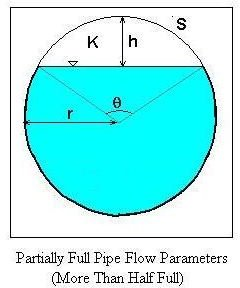The Excel template shown at the right can be used to calculate the volumetric flow rate, Q, and flow velocity, V,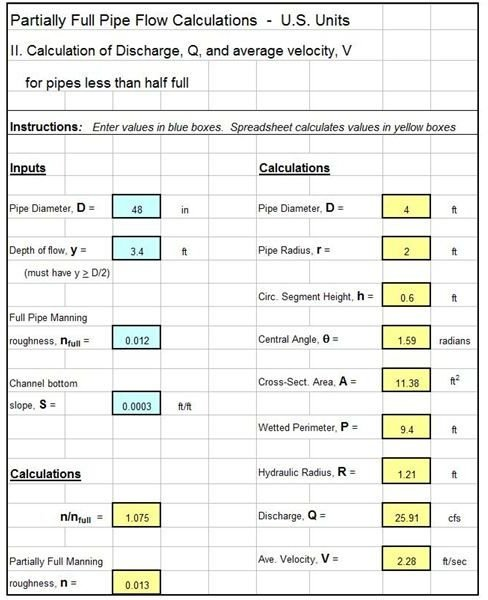for specified values of D, nfull, and S for a partially full pipe that is flowing more than half full.

The diagram at the left shows the parameters used in the previous section as they apply to partially full pipe flow with depth more than half of the pipe diameter. The same parameters, h, S, θ, and r are shown as they will be used for this flow situation.

The equations to be used for this case of flow depth more than pipe radius are shown below and are used for the Excel formulas in the spreadsheet template.

• h = 2r - y
• θ = 2 arccos[ (r - h)/r ]
• A = πr2 - K = πr2 - r2(θ - sinθ)/2
• P = 2πr - S = 2πr - rθ

The equation used for n/nfull for 0.5 < y//D < 1 is: n/nfull = 1.25 - [(y/D - 0.5)/2]

The third page of this article presents and discusses spreadsheet templates for calculation of normal depth in partially full pipe flow.

## Normal Depth Calculation for Pipes Flowing Less Than Half Full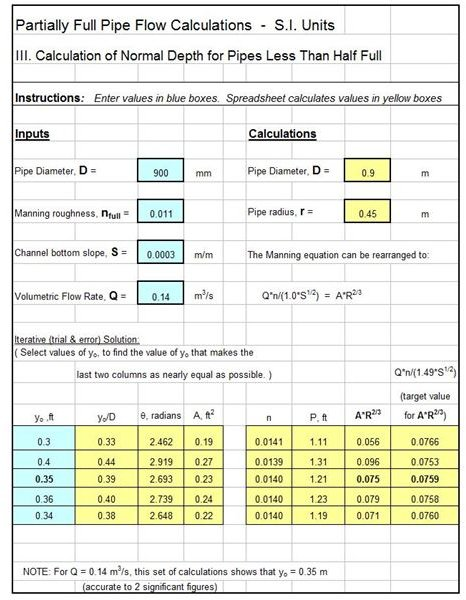The Excel template shown at the left can be used to calculate the normal depth, yo, for specified pipe diameter, D; full pipe Manning roughness, nfull; pipe bottom slope, S; and volumetric flow rate, Q, for a partially full pipe that is flowing less than half full.

The diagram and equations given in the third section (page 2 of this article) for partially full pipe flow with flow depth less than half of the pipe diameter apply here also. The determination of normal depth is an iterative (trial and error) calculation for this situation, just as it is for a rectangular channel or a trapezoidal channel.

In this case of partially full pipe flow, for each assumed value of flow depth, yo, it is necessary to calculate h, A, P, R, and n, so that the parameter, AR2/3 can be calculated. Assuming values of yo and calculating values for AR2/3, allows one to zero in on the yo value that comes closest to the target value for AR2/3, as shown in the Excel template example. The example calculation in this spreadsheet template resulted in yo = 0.35 m, accurate to 2 significant figures.

Note that if the answer comes out to be more than the pipe radius, then the spreadsheet template in the next section should be used, because it uses the slightly different equations that apply for depth greater than the pipe radius.

## Normal Depth Calculation for Pipes Flowing More Than Half Full

The Excel template shown at the right can be used to calculate the normal depth, yo, for specified pipe diameter, D; full pipe Manning roughness,nfull; pipe bottom slope, S; and volumetric flow rate, Q, for a partially full pipe that is flowing more than half full.

The diagram and equations given in the last section on page 2 of this article, for partially full pipe flow with flow depth more than half of the pipe diameter, apply here also. The table of iterative calculations in this spreadsheet template is much the same as in the last section, but uses the slightly different equations that apply for depth of flow greater than the pipe radius. The example calculation in this spreadsheet template resulted in yo= 0.57 m, accurate to 2 significant figures.

Note that if the answer comes out to be less than the pipe radius, then the spreadsheet template in the previous section should be used, because it uses the slightly different equations that apply for depth less than the pipe radius.

## Summary

This article discussed four Excel spreadsheet templates for making Manning Equation/uniform open channel flow calculations for the particular case of partially full pipe flow. For more general information about the Manning equation and its use for uniform open channel flow, see the articles in the series, “Uniform Open Channel Flow and the Manning Equation,” for example through the article, “Introduction to the Manning Equation for Uniform Open Channel Flow Calculations.” Excel spreadsheet templates for several other types of open channel flow calculations can be accessed through the articles in that series.

## References and Image Credits

References for further information:

1. Bengtson, Harlan H., Open Channel Flow I - The Manning Equation and Uniform Flow, an online, continuing education course for PDH credit.

2. Camp, T.R., “Design of Sewers to Facilitate Flow,” Sewage Works Journal, 18 (3), 1946

3. Chow, V. T., Open Channel Hydraulics, New York: McGraw-Hill, 1959.

4. Steel, E.W. & McGhee, T.J., Water Supply and Sewerage, 5th Ed., New York, McGraw-Hill Book Company, 1979

Images were all drawn/prepared by H. Bengtson